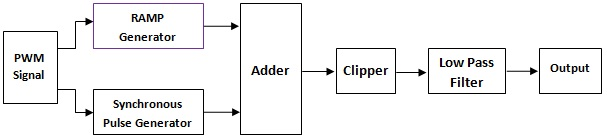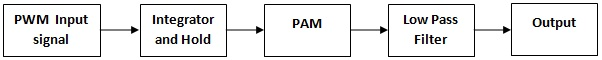Pulse Width Demodulation

Objective
To study the and implementing a Pulse Width Demodulator.

Introduction

The square generator and the monostable multivibrator circuits were used to generate the PWM signal. To recover the original audio signal from a PWM signal, a decoder or demodulator is need in the receiver circuit.
There are two common techniques used for pulse-width demodulation. One method is that the PWM signal must first be converted to a pulse-amplitude modulation (PAM) signal and then passed through a low-pass filter. The PWM signal is applied to an integrator and hold circuit. When the positive edge of pulse appears, the integrator generates ramp output whose magnitude is proportional to the pulse width. After the negative edge, the hold circuit maintains the peak ramp voltage for a given period and then forces the output voltage to zero. The waveform is the sum of a sequence of constant-amplitude and constant-width pulse generated by demodulator. This signal is then applied to the input of clipping circuit, which cuts off the portion of signal below the threshold voltage and outputs the reminder. Therefore the output of clipping circuit is a PAM signal whose amplitude is proportional to the width of PWM signal. Finally, the PAM signal passes through a simple low-pass filter and the original audio signal is obtained.Fig.1 Shows the schematic diagram of PWM Demodulation.

The other technique for demodulating a PWM signal is consist of a product detector and a low-pass filter. The PWM and the carrier signals are connected to the inputs of a product detector, and then a sequence of pulses having the width inversely proportional to the width of PWM pulse presents at output. When the Va signal passes through the low-pass filter, a demodulated signal is obtained.Fig.2 Shows the schematic diagram of another scheme for PWM Demodulation.

For any pulse wave modulation, before modulating, the original continuous type signal must be sampled and the sampling rate of the sampling signal cannot be low, or else the recovered signal will cause distortion. The sampling rate depends on the sampling theorem which the sampling theorem is defined as: for any pulse wave modulation system, if the sampling rate excesses double or more times of the maximum frequency of the signal, then the distortion level of the data recovery at the receiver will be the minimum. For example, the frequency range of the audio signal is 40 Hz ~ 4 kHz, then the sampling signal frequency of the pulse wave modulation must be at least 8 kHz, therefore, the sampling error can be reduced to minimum.